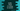# Kotlin program to check if a string is numeric## Kotlin check if a string is numeric :

In this post, I will show you how to check if a string is numeric or not in Kotlin. For example, “1.2” is a numeric string but 1.2x is not. We will learn different ways to solve this problem.

### Method 1: Using string.toDouble() :

Kotlin provides a couple of functions for the string class. We can parse a string as an integer or as a double. toInt() method parse one string as an integer and returns the result. Similarly, toDouble parse one string as Double.

For our problem, we will try to parse the string as double i.e. we will use toDouble method. This method throws one exception, NumberFormatException if the parsing fails. So, for a string, if this method can parse its value, we can say that this string is numeric. Take a look at the below example program :

``````fun isNumber(str: String) = try {
str.toDouble()
true
} catch (e: NumberFormatException) {
false
}

fun main() {
val strArray = arrayOf("1", "-2", "0", "1.2", "-1.2", "xy", "+-1.2")

strArray.forEach {
println("\$it => \${isNumber(it)}")
}
}``````

Here, isNumber method takes one String as argument and returns one boolean. In the try block, it tries to convert the string to a double using toDouble method. If the conversion is successful, i.e. if it is a number, it returns true. Else, it catches one exception and returns false.

The above program will print the below output :

``````1 => true
-2 => true
0 => true
1.2 => true
-1.2 => true
xy => false
+-1.2 => false``````

### Method 2: Using toDoubleOrNull :

toDoubleOrNull is another version of toDouble. It converts one string to double, if the conversion is successful, it returns that converted value and if it fails, it returns null. So, we don’t have to use any try-catch block here :

``````fun isNumber(str: String) = str.toDoubleOrNull()?.let { true } ?: false

fun main() {
val strArray = arrayOf("1", "-2", "0", "1.2", "-1.2", "xy", "+-1.2")

strArray.forEach {
println("\$it => \${isNumber(it)}")
}
}``````

It will print the same output as the above program. Simpler and cleaner.